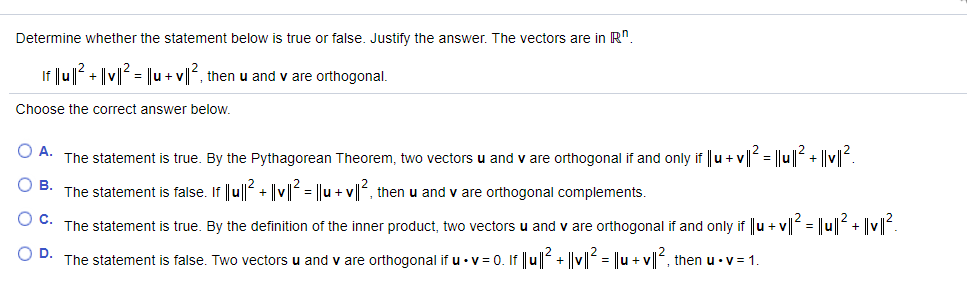# Determine whether the statement below is true or false. Justify the answer. The vectors are in R". If Ju|? + |v| = ||u +v|l°, then u and v are orthogonal. Choose the correct answer below. A. The statement is true. By the Pythagorean Theorem, two vectors u and v are orthogonal if and only if u+ v =D В. The statement is false. If ||u|| + ||v| = ||u + v||, then u and v are orthogonal complements. с. The statement is true. By the definition of the inner product, two vectors u and v are orthogonal if and only if u + v||² = ||u|2+ |v|. D. The statement is false. Two vectors are orthogonal if u · v = 0. If |lu|? + ||v|? = |u + v|?, then u - v = 1. and %3D O O O O

Question

Determine whether the statement below is true or false. Justify the answer. The vectors are in Rn. If (see picture), then u and v are orthogonal.help_outlineImage TranscriptioncloseDetermine whether the statement below is true or false. Justify the answer. The vectors are in R". If Ju|? + |v| = ||u +v|l°, then u and v are orthogonal. Choose the correct answer below. A. The statement is true. By the Pythagorean Theorem, two vectors u and v are orthogonal if and only if u+ v =D В. The statement is false. If ||u|| + ||v| = ||u + v||, then u and v are orthogonal complements. с. The statement is true. By the definition of the inner product, two vectors u and v are orthogonal if and only if u + v||² = ||u|2+ |v|. D. The statement is false. Two vectors are orthogonal if u · v = 0. If |lu|? + ||v|? = |u + v|?, then u - v = 1. and %3D O O O O fullscreen

### Want to see this answer and more?

Experts are waiting 24/7 to provide step-by-step solutions in as fast as 30 minutes!*

*Response times may vary by subject and question complexity. Median response time is 34 minutes for paid subscribers and may be longer for promotional offers.
Tagged in
Math